# Thermo-hydraulic performance analysis of jet plate solar air heater under cross flow condition

Thermo-hydraulic performance analysis of jet plate solar air heater under cross flow condition

Prashil D. Vinod Shailendra N. Singh*

Heat Transfer Laboratory, Department of ME, Indian Institute of Technology (ISM), Dhanbad, Jharkhand 826004

Corresponding Author Email:
snsingh631@yahoo.com
Page:
603-610
|
DOI:
https://doi.org/10.18280/ijht.350317
|
Accepted:
|
Published:
30 September 2017
| Citation

OPEN ACCESS

Abstract:

In order to transfer higher heat from absorber plate to flowing air stream to increase the amount of collected energy and thus, to improve efficiency of solar air heater, a jet impingement concept has been studied. To investigate the effect of geometrical parameters like hole configuration on the jet plate i.e., inline and staggered holes, and operational parameter like velocity of air impinging out of the holes on to surface of the absorber plate on the performance of jet plate solar air heater, a detailed analysis has been performed on the design for different air mass flow rates. Experiments are performed with air mass flow rates, ṁ1= 0.05-0.105 kg/sec, ṁ2 = 0.033-0.07 kg/sec, Re = 0.59×104-1.15×104, jet hole diameter, D = 0.006 m. Also, a parallel study has been made on a conventional parallel plate solar air heater to have comparison of air temperature increment and collector efficiency with those of the jet plate solar heater. The results obtained from the present study show that the mass flow rate of air influences heat transfer in the jet plate solar air heater. The performance of cross flow solar air heater with inline hole jet plate is superior over that with staggered hole jet plate.

Keywords:

jet plate, collector efficiency, absorber plate, convective heat transfer coefficient, nusselt number, friction factor

1. Introduction

A solar air heater is an important solar energy collecting system that can be extensively used in the fields of space heating, drying of agriculture crops and supply of hot air in buildings . The thermal efficiency of the solar air heater is generally low due to its low convective heat transfer coefficient between the absorber plate and the flowing air that increases absorber plate temperature leading to higher heat loss to the ambient. For improving the thermal performance of the heater, heat must be transferred efficiently.

The jet impingement concept is one of the effective methods for increasing the heat transfer in a solar air heater. In the past, a conventional solar air heater has been modified in order to increase heat transfer coefficient between absorber plate and air flowing over it. Choudhury and Garg evaluated a gain in air temperature increment and performance efficiency of the cross and non-cross flow inline hole jet concept air heater over that of the parallel plate air heater with duct depth 10.0 cm and length 2.0 m is 15.5 °C to 2.5 °C and 26.5 % to 19.0 % respectively, for air flow rates in the range 50.0 to 250.0 kg/hm2 . Kercher and Tabakoff conducted an experiment on jet plate solar air heater and derived a correlation for Nusselt number . Metzger et. al. studied heat transfer characteristics for inline and staggered arrays of circular jets with cross ‐ flow spent air . Singh presented heat transfer enhancement in a continuous longitudinal fins solar air heater for different pitches analytically . Thombre and Sukhatme studied the variation over conventional solar air heater with longitudinal fins fitted under the lower surface of the absorber plate .  Xing et al. presented experimental and numerical results on heat transfer characteristics of inline and staggered hole jet plate in cross flow condition . Singh investigated flow and heat transfer in a double pass counter flow solar air heater . Chauhan and Thakur experimentally investigated and correlated the effect of geometrical parameters (i.e. the jet hole diameter, stream‐wise and span‐wise pitch of the jet holes) on Nusselt number and friction factor for impinging jet solar air heater . Nayak and Singh studied the performance of a cross-flow staggered hole jet plate solar air heater . Soni and Singh analyzed the effect of geometrical parameters on the performance of inline hole jet plate solar air heater under cross flow condition .

From the above cited literature, it is clear that sufficient work has been performed on cross flow jet plate solar air heater with inline hole. Also, a little amount of literature is available on the performance of cross flow jet plate solar air heater with staggered hole. Hence, the present work is performed in a need to investigate and compare the behavior of cross flow jet plate solar air heater with inline and staggered hole experimentally which was not analyzed by Choudhury and Garg . The present investigation is aimed to study and compare heat transfer characteristic and collector efficiency of jet plate solar air heater with inline and staggered holes. The results are also compared with conventional parallel plate solar air heater without jet plate.

2. Set Up Description and Procedure

2.1 Description of the experimental set up and procedure

Figure 1 represents cross-sectional view of conventional parallel plate solar air heater having total air depth Z. Figure 2 shows the schematic cross-sectional view of cross flow jet plate solar air heater with inline and staggered holes with air flowing in bottom and upper channel having depth Z1 and Z2.

## 1.png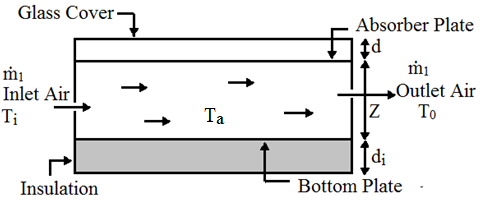Figure 1. Schematic cross-sectional view of conventional parallel solar air heater

## 2.png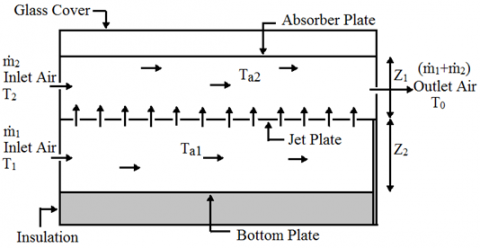Figure 2. Schematic cross-sectional view of cross flow jet plate solar air heater

The photographic view of the jet plate solar air heater is shown in figure 3. It consists of blower, toughened glass cover plate, black painted absorber plate, jet plate, bottom plate, toughened glass cover plate, voltage regulator, three digital temperature display units (DTDU), thermocouples embedded on each plate. The sides and bottom of the air heater are properly insulated with glass wool. The photographic views of jet plates with inline and staggered holes are shown in figure 4 and figure 5, respectively.

In the present study, cross flow jet plate solar air heater is converted into a conventional parallel plate solar air heater by removing jet plate from the system. In the jet plate air heater, the inlet air at bottom channel (ṁ1) and upper channel (ṁ2) are supplied by a single blower regulated by a voltage regulator. The air with mass flow rate ṁ1 impinging out of the hole is mixed with ṁ2 and comes out from the upper channel as shown in figure 2. Digital Hot Wire Anemometer (DHWA) measures the inlet velocity V1 and temperature T1 of air at the bottom channel and, inlet velocity V2 and temperature T2 of air at the upper channel whereas the same measures outlet velocity V0, and outlet temperature T0 of the mixed air ṁ1 and ṁ2 at the exit of the upper channel. Temperatures of the upper and lower parts of the absorber plate, upper and lower part of the jet plate and bottom plate are measured with thermocouples fitted at different local points of the absorber plates, jet plate and bottom plate of the air heater. All the temperatures are read by digital temperature display unit, DTDU. The same methods are used for measuring the absorber plate temperature, bottom plate temperature and inlet velocity (V1), inlet temperature (Ti), outlet velocity (V0) and outlet temperature (T0) of air ṁ1 in the parallel plate solar air heater. The ambient temperature (Ta) is measured by DHWA and the solar intensity is recorded by a Digital solar intensity recorder. The uncertainties of various instruments used are listed in the table 1.

## 3.pngFigure 3. Photographic view of experimental set up

## 4.png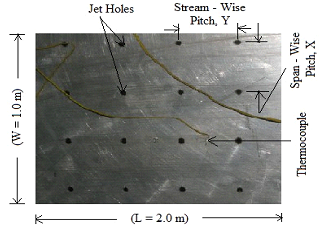Figure 4. Photographic view of jet plate with inline hole

## 5.png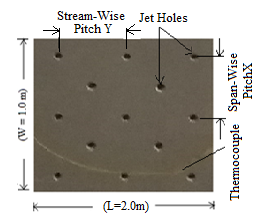Figure 5. Photographic view of jet plate with staggered hole

Experiments have been conducted during winter season with clear sunshine in the months of January-February 2017 at IIT(ISM), Dhanbad (Jharkhand), India. All the test readings have been recorded on hourly basis in between 9.00 a.m. to 3.00 p.m.

Table 1. Instruments with their uncertainties

 Sr.No. Name of the instrument Uncertainty 1. Digital Hot Wire Anemometer ± 0.05 m/s  (velocity) ± 0.8 °C (temperature) 2. Thermocouple ± 2.2 °C 3. Digital solar intensity recorder ± 0.25 W/m2

2.2 Specifications of experimental set up

All the specifications of the experimental set up have been listed in Table 2.

Table 2. Specifications of Experimental Set up

 Sr.No. Nomenclature Specifications 1. Length of solar air heater L = 2.0 m 2. Width of collector W = 1.0 m 3. Spacing between bottom and jet plate Z1 = 0.078 m 4. Spacing between absorber and jet plate Z2 = 0.078 m 5. Absorber plate t = 1.0 mm, α = 0.95 6. Jet plate t = 4.0 mm, Al-alloy material 7. Diameter of jet hole D = 0.006 m 8. Number of holes on inline hole jet plate N = 561 9. Number of holes on staggered hole jet plate N = 1173 10. Glass cover t = 4.0 mm, τ = 0.95 11. Tilt angle θ = 22.6° 12. Insulation t = 0.025 m, Glass wool material
3. Data Reduction

The collected experimental data are used for computing the outlet air temperature, collector efficiency, heat transfer coefficient, Nusselt number and friction factor in order to determine the effect of jet impingement on the heat transfer characteristics of the solar air heater. Under steady state condition, the following equations are used:

Convective heat transfer coefficient of absorber plate to jet air for cross flow jet plate solar air heater with inline and staggered holes,

$\mathrm{h}_{\mathrm{pj}}=\frac{\left(\dot{\mathrm{m}}_{1}+\dot{\mathrm{m}}_{2}\right) \mathrm{C}\left(\mathrm{T}_{0}-\mathrm{T}_{\mathrm{i}}\right)}{\mathrm{A}_{\mathrm{p}}\left(\mathrm{T}_{\mathrm{p}}-\mathrm{T}_{\mathrm{a} 2}\right)}$     (1)

where, temperature of inlet air above jet plate in mixing of air,

$\mathrm{T}_{\mathrm{i}}=\frac{\mathrm{m}_{1} \mathrm{T}_{1}+\mathrm{m}_{2} \mathrm{T}_{2}}{\mathrm{m}_{1}+\mathrm{m}_{2}}$

and temperature of air in upper channel,

$\mathrm{T}_{\mathrm{a} 2}=\frac{\left(\dot{\mathrm{m}_{1}} \mathrm{T}_{1}+\dot{\mathrm{m}_{2}} \mathrm{T}_{2}\right)+\left(\dot{\mathrm{m}_{1}}+\mathrm{m}_{2}\right) \mathrm{T}_{0}}{2\left(\dot{\mathrm{m}_{1}}+\dot{\mathrm{m}}_{2}\right)}$

Collector efficiency of cross flow jet plate solar air heater with inline and staggered holes,

$\eta_{\mathrm{c}}=\frac{\left(\dot{\mathrm{m}_{1}}+\dot{\mathrm{m}}_{2}\right) \mathrm{C}\left(\mathrm{T}_{0}-\mathrm{T}_{\mathrm{a}}\right)}{\mathrm{A}_{\mathrm{p}} \mathrm{I}}$     (2)

Reynolds number in upper channel of cross flow jet plate solar air heater with inline and staggered holes,

$\operatorname{Re}_{\mathrm{pj}}=\frac{\rho \mathrm{VD}_{2}}{\mu}$     (3)

where, average velocity of air in upper channel,

$\mathrm{V}=\frac{\left(\mathrm{V}_{\mathrm{av}}+\mathrm{V}_{0}\right)}{2}$,

average velocity of jet air and inlet velocity in upper channel,

$\mathrm{V}_{\mathrm{av}}=\frac{\mathrm{A}_{\mathrm{j}} \mathrm{V}_{\mathrm{j}}+\mathrm{A}_{2} \mathrm{V}_{2}}{\mathrm{A}_{\mathrm{j}}+\mathrm{A}_{2}}$,

cross sectional area of upper channel,

$\mathrm{A}_{2}=\mathrm{WZ}_{2}$,

velocity of jet air,

$\mathrm{V}_{\mathrm{j}}=\frac{4 \mathrm{m}_{1}}{\rho \pi \mathrm{D}^{2} \mathrm{N}}$,

hydraulic diameter of upper channel,

$\mathrm{D}_{2}=\frac{4 \mathrm{WZ}_{2}}{2\left(\mathrm{W}+\mathrm{Z}_{2}\right)}$

Nusselt number in upper channel of cross flow jet plate solar air heater with inline and staggered holes,

$\mathrm{Nu}_{\mathrm{pj}}=\frac{\mathrm{h}_{\mathrm{p}} \mathrm{D}_{2}}{\mathrm{K}_{\mathrm{a}}}$     (4)

where, jet Reynolds number,

$\operatorname{Re}_{\mathrm{D}}=\frac{\rho \mathrm{V}_{\mathrm{j}} \mathrm{D}}{\mu}$.

It is assumed that Vj is uniform throughout all the holes.

The Nusselt number for smooth rectangular duct is given by Dittus–Boelter equation as

$\mathrm{Nu}_{\mathrm{pj}}=0.023\left(\mathrm{Re}_{\mathrm{pj}}\right)^{0.8}(\mathrm{Pr})^{0.4}$     (5)

Friction factor in the upper channel of cross flow jet plate solar air heater with inline and staggered holes,

$\mathrm{f}=\frac{\Delta \mathrm{pD}_{2}}{2 \rho \mathrm{LV}^{2}}$     (6)

where, pressure drop in the flow channel,

$\Delta \mathrm{p}=\frac{32 \mu \mathrm{VL}}{\mathrm{D}_{2}^{2}}$

The friction factor for a smooth rectangular duct is given by modified Blassius equation as

$\mathrm{f}=0.085\left(\mathrm{Re}_{\mathrm{pj}}\right)^{-0.25}$     (7)

Instantaneous efficiency of cross flow jet plate solar air heater with inline and staggered holes,

$\eta_{\mathrm{i}}=\mathrm{F}_{\mathrm{R}}\left[\tau \alpha-\mathrm{U}_{1} \frac{\left(\mathrm{T}_{\mathrm{a} 2}-\mathrm{T}_{\mathrm{a}}\right)}{\mathrm{I}}\right]$     (8)

In case of conventional parallel plate solar air heater without jet plate, the coefficient of heat transfer from absorber plate -to- air stream,

$\mathrm{h}_{\mathrm{pa}}=\frac{\dot{\mathrm{m}_{1}} \mathrm{C}\left(\mathrm{T}_{0}-\mathrm{T}_{a}\right)}{\mathrm{A}_{\mathrm{p}}\left(\mathrm{T}_{\mathrm{p}}-\mathrm{T}_{\mathrm{A}}\right)}$     (9)

Nusselt number in the flow channel of parallel plate solar air heater,

$\mathrm{Nu}_{\mathrm{pa}}=\frac{\mathrm{h}_{\mathrm{pa}} \mathrm{D}_{\mathrm{h}}}{\mathrm{K}_{\mathrm{a}}}$     (10)

where, hydraulic diameter for parallel plate air heater,

$\mathrm{D}_{\mathrm{h}}=\frac{4 \mathrm{WZ}_{1}}{2\left(\mathrm{W}+\mathrm{Z}_{1}\right)}$

Reynolds number in the flow channel of parallel plate solar air heater,

$\operatorname{Re}_{\mathrm{pa}}=\frac{\rho \mathrm{VD}_{\mathrm{h}}}{\mu}$     (11)

Collector efficiency of parallel plate solar air heater,

$\eta_{\mathrm{c}}=\frac{\dot{\mathrm{m}_{1}} \mathrm{C}\left(\mathrm{T}_{0}-\mathrm{T}_{\mathrm{a}}\right)}{\mathrm{A}_{\mathrm{p}} \mathrm{I}}$     (12)

The various thermo-physical properties of air used in the calculations were obtained from a text by Duffie and Beckman .

4. Uncertainty Analysis

Kline and McKlintock method  has been used for finding the uncertainty:

For the case of cross flow inline hole jet plate solar air heater, the maximum uncertainty of ±8.98 % is obtained in heat transfer coefficient (hpj). The individual errors contributed to the uncertainty of heat transfer coefficient for the measured and physical properties are: mass flow rate in bottom channel (ṁ1): ±5.02 %, mass flow rate in upper channel (ṁ2): ±8.1 %, absorber plate temperature (Tp): ±2.21 %, outlet air temperature (T0): ±3.21 %, average of the inlet air temperature above jet plate (Ti): ±1.22 %, and temperature of air in upper channel (Ta2): ±2.15 %. The maximum uncertainties of non-dimensional parameters are: Reynolds number (Reja2): ±4.33%, Nusselt number (Nuja2): ±8.98 % and friction factor (f): ±4.16 %

Similarly, for cross flow staggered hole jet plate solar air heater, the maximum uncertainty of ±9.38 % is obtained in heat transfer coefficient (hpj). The individual errors contributed to the uncertainty of heat transfer coefficient for the measured and physical properties are: mass flow rate in bottom channel (ṁ1): ±6.17 %, mass flow rate in upper channel (ṁ2): ±4.33 %, absorber plate temperature (Tp): ±2.21 %, outlet air temperature (T0): ±3.21 %, average of the inlet air temperature above jet plate (Ti): ±2.17 %, and temperature of air in upper channel (Ta2): ±2.23 %. The maximum uncertainties of non-dimensional parameters are: Reynolds number (Reja2): ±5.02%, Nusselt number (Nuja2): ±9.38 % and friction factor (f): ±6.01 %.

5. Results and Discussion

5.1 Validation

## 6.png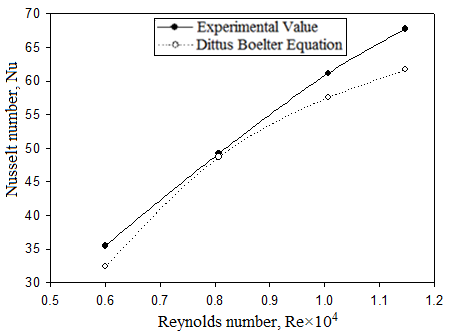Figure 6. Comparison of experimental and predicted values of Nusselt number for air heater with inline holes

## 7.png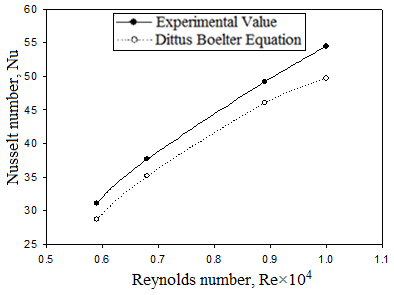Figure 7. Comparison of experimental and predicted values of Nusselt number for air heater with staggered holes

The validity tests have been carried out to serve as a basis of comparison of results with the values of Nusselt number and friction factor from the correlations for the smooth rectangular duct stated in eq. (5) and eq. (7), respectively.

The comparison of experimental and predicted values from Dittus Boelter equation of Nusselt number as function of Reynolds number for the air heater with inline hole jet plate and staggered hole jet plate have been shown in figure 6 and figure 7, whereas comparison of experimental and predicted values from Blassius equation of friction factor as a function of Reynolds number for air heater with inline hole jet plate and staggered hole jet plate have been shown in figure 8 and figure 9, respectively. The average absolute deviation of the present experimental Nusselt number for air heater with inline hole jet plate and staggered hole jet plate are 6.15 % and 7.39 % from the values predicted by eq. (11) and average absolute deviation of friction factor for air heater with inline hole jet plate and staggered hole jet plate are 5.51 % and 8.88 % from the values predicted by eq.(12). This shows a good agreement between experimental and predicted values, which ensures the accuracy of the experimental data collected for investigation.

## 8.png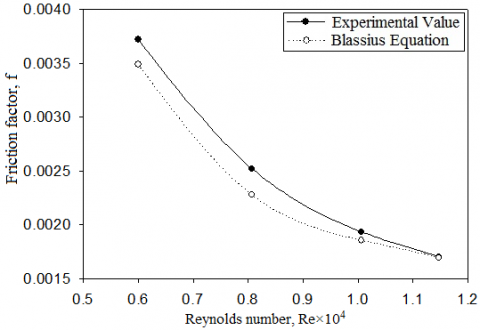Figure 8. Comparison of experimental and predicted values of friction factor for air heater with inline holes

## 9.png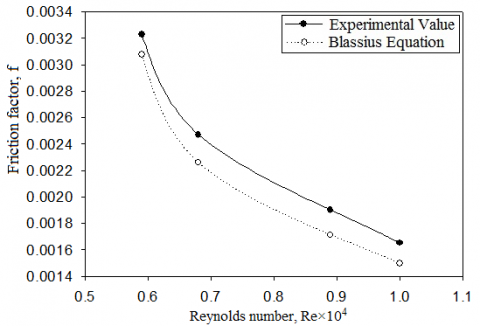Figure 9. Comparison of experimental and predicted values of friction factor for air heater with staggered holes

5.2 Typical experimental results

The experimental data used to study various heat transfer characteristics are discussed as follows:

5.2.1 Effect of mass flow rate of air on outlet air temperature and collector efficiency

## 10.png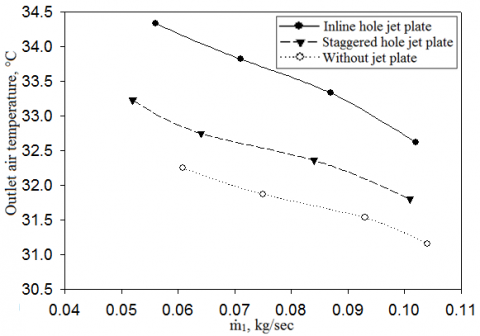Figure 10. Variation of outlet air temperature with mass flow rate of air

## 11.pngFigure 11. Variation of collector efficiency with mass flow rate of air

Figure 10 and figure 11 show variation of outlet air temperature and collector efficiency, respectively of the cross-flow jet plate solar air heater with inline and staggered holes for different mass flow rate of air. Outlet air temperature of solar air heater decreases whereas, collector efficiency increases with increasing mass flow rate (ṁ1) of air. For the same jet hole diameter, as the hole arrangement in the jet plate is changed from inline holes to staggered holes, the values of outlet air temperature and collector efficiency are reduced. For the fixed mass flow rate of air and channel width, the velocity of the jet coming out of the holes of jet plate has higher value for inline hole jet plate than that for staggered hole jet plate. As the air coming out of the holes in the form of jet strike the lower surface of the absorber plate thus resulting in the increment in the heat transfer coefficient, the collector efficiency of the cross-flow jet plate solar air heater with inline hole jet plate has higher values than that with staggered hole jet plate. The maximum gains in the outlet air temperature and collector efficiency of the solar air heater with inline hole jet plate are found to be 1.4 °C and 4.6 % respectively as compared to those with staggered hole jet plate. Here, with increase in the mass flow rate (ṁ1) of air, the increment in the outlet air temperature is lower whereas increment in the collector efficiency is higher due to addition of the cross-flow air (ṁ2). The performance of the jet plate solar air heaters is found to be improved due to larger coefficient of heat transfer from absorber plate to air in the system than that in the conventional parallel plate solar air heater without jet plate. For the fixed mass flow rate (ṁ1), the performance of the conventional parallel plate solar air heater is lower due to lower values of Reynolds number (Repa).

5.2.2 Effect of Reynolds number on nusselt number

Figure 12 shows the variation of Nusselt number with Reynolds number for cross flow solar air heater with inline hole jet plate and staggered hole jet plate, and conventional parallel plate solar air heater without jet plate. For fixed values of Reynolds number, the Nusselt number for solar air heater with inline hole jet plate is found higher than that with staggered hole jet plate. For the same diameter of jet hole, the air coming out of the jet holes impinge on to lower surface of the absorber plate at higher velocity in case of air heater with inline hole jet plate than that with staggered hole jet plate. This results in the increase in the heat transfer coefficient (hpj) and thus in the Nusselt number for inline hole air heater. The maximum increment in the Nusselt number for inline hole jet plate solar air heater is found to be 15.4 % higher than that for staggered hole jet plate solar air heater. In case of conventional parallel plate solar air heater without jet plate, the depth of channel (Z) is higher as compared depth of channel (Z2) in cross flow jet plate solar air heater. Due to increased channel depth, flow Reynolds number Repa decreases for fixed mass flow rate (ṁ1) of air leading to lower value of Nusselt number in parallel plate solar air heater. For fixed Reynolds number, the maximum increment in Nusselt number in cross flow inline hole jet plate solar heater is found to be 31.9 % higher than that for parallel plate solar air heater without jet plate.

## 12.png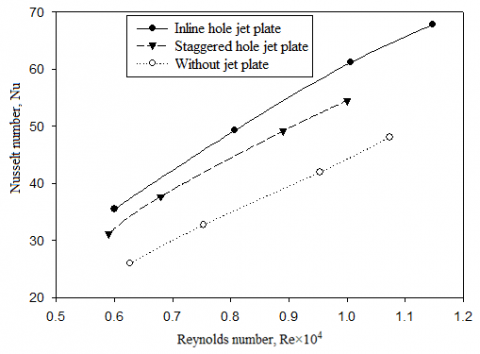Figure 12. Variation of Nusselt number with Reynolds number for solar air heater with inline and staggered hole jet plates and conventional parallel plate solar air heater

5.2.3 Effect of Reynolds number on friction factor

Figure 13 shows the variation of friction factor with Reynolds number for cross flow solar air heater with inline hole jet plate and staggered hole jet plate, and conventional parallel plate solar air heater without jet plate. From the figure, it is seen that the friction factor decreases as Reynolds number increases for both jet plate solar air heater and conventional parallel plate solar air heater. For fixed Reynolds number, friction factor for cross flow air heater with inline hole jet plate is found to have higher values than those with staggered hole jet plate. These higher values of friction factor result into higher pressure drop in the upper channel of inline hole jet plate air heater. It is seen from the Darcy Weisbach formula, that the pressure drop is directly proportional to the square of flow velocity in the channel and inversely proportional to the hydraulic diameter of the flow channel. Because of higher value of hydraulic diameter (Dh) of the parallel plate solar air heater than the hydraulic diameter (D2) of the cross-flow jet plate air heater, the pressure drop in the parallel plate air heater is lower as compared to cross flow jet plate air heater.

## 13.png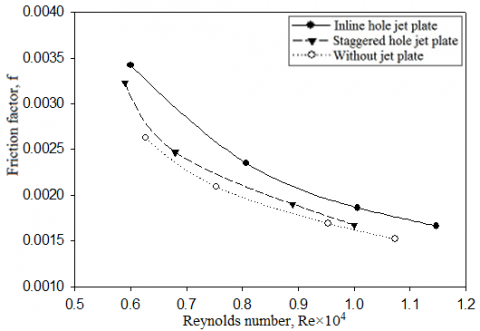Figure 13. Variation of friction factor with Reynolds number for solar air heater with inline and staggered hole jet plates and conventional parallel plate solar air heater

5.2.4 Variation of instantaneous efficiency with $\frac{\left(\mathrm{T}_{\mathrm{a} 2}-\mathrm{T}_{\mathrm{a}}\right)}{\mathrm{I}}$ and mass flow rate

## 14.png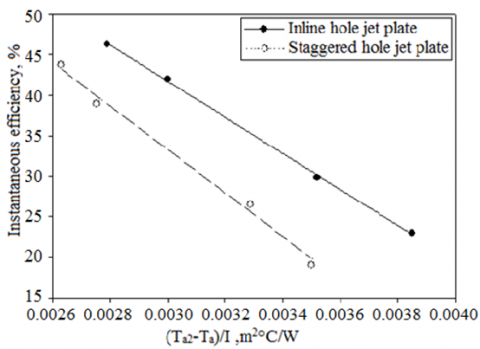Figure 14. Variation of Instantaneous efficiency with $\frac{\left(\mathrm{T}_{\mathrm{a} 2}-\mathrm{T}_{\mathrm{a}}\right)}{\mathrm{I}}$ and mass flow rate for solar air heater with inline hole and staggered hole jet plates

Figure 14 shows variation of instantaneous efficiency of the jet plate solar air heater for different mass flow rate of air with factor$\frac{\left(\mathrm{T}_{\mathrm{a} 2}-\mathrm{T}_{\mathrm{a}}\right)}{\mathrm{I}}$. The figure shows linear behavior with negative slope FRUl and intercept FR(τα), giving the heat transfer characteristics of the jet plate solar air heater. The negative slope of the lines represent the rate of heat loss from the solar air heater to the atmosphere. It is seen from the figure that as the factor $\frac{\left(\mathrm{T}_{\mathrm{a} 2}-\mathrm{T}_{\mathrm{a}}\right)}{\mathrm{I}}$ decreases, instantaneous efficiency increases for different mass flow rates of air. This shows that the useful heat gain rate for the collector can be increased by either increasing the intensity of solar radiation or increasing the mass flow rate of air through the channel. The instantaneous efficiency of the cross-flow air heater with inline hole jet plate is found to be higher than that with staggered hole jet plate.

6. Conclusions

The performance of cross flow solar air heater with inline hole jet plate and with staggered hole jet plate have been investigated experimentally and compared for various mass flow rate of air. It can be seen from the results that the outlet air temperature, collector efficiency and heat transfer in the inline hole jet plate solar air heater are significantly higher while comparing with those in staggered hole jet plate solar air heater. The maximum percentage gain in outlet air temperature increment, collector efficiency and Nusselt number of the cross flow solar air heater with inline hole jet plate over those with staggered hole jet plate for channel depth of 0.078 m and length of 2 m are 4.26 %, 4.6 % and 15.4 % respectively. However, there is no significant change in the friction factor seen in the work. Therefore, it can be concluded that performance of solar air heater with inline hole jet plate is superior over that with staggered hole jet plate. Moreover, the results are compared with the conventional parallel plate solar air heater without jet plate proving the superiority in the performance of jet plate solar air heater that mentioned in the literature.

Nomenclature
 Ap surface area of absorber plate, m2 Aj area of jet hole, m2 A2 cross – sectional area of upper channel for jet plate solar air heater, m2 C specific heat capacity of air, kJ/kgK d distance between absorber and glass cover, m di insulation thickness, m D diameter of jet hole, m Dh hydraulic diameter for conventional parallel plate solar air heater, m D2 hydraulic diameter of upper channel for jet plate solar air heater, m f friction factor FR heat removal factor hpa plate to air heat transfer coefficient in parallel plate air heater, W/m2 hpj average plate‐to‐jet air heat transfer coefficient for jet plate solar air   heater, W/m2 I incident solar intensity, W/m2 Ka thermal conductivity of air flowing through duct, W/mK L length of air heater, m m dimensionless number ṁ1 mass flow rate of air in parallel plate air heater and through bottom   channel in jet plate air heater, kg/sec ṁ2 mass flow rate of cross ‐ flow air through upper channel for jet plate solar   air heater, kg/sec N total number of jet holes on jet plate Nupj Nusselt number between absorber plate and jet plate for jet plate solar air   heater Nupa Nusselt number between absorber plate and bottom plate for parallel   plate air heater Pr Prandtl number Δp pressure drop, N/m2 Rej jet Reynolds number for jet plate solar air heater Repj flow Reynolds number between absorber plate and jet plate for jet plate   solar air heater Repa flow Reynolds number between absorber plate and bottom plate for   parallel plate air heater Ta channel air temperature in parallel plate air heater, °C T1 inlet air temperature at bottom channel for jet plate solar air heater, °C T2 inlet air temperature at upper channel for jet plate solar air heater, °C TA ambient temperature, °C Ta1 air temperature at lower channel for jet plate solar air heater, °C Ta2 air temperature at upper channel for jet plate solar air heater, °C Ti inlet air temperature of parallel plate solar air heater and inlet air   temperature above jet plate in mixing of air for jet plate solar air heater, °C T0 outlet air temperature, °C Tp absorber plate temperature, °C Ul total heat loss coefficient, W/m2°C V1 wetted mean inlet velocity of air in the lower channel for jet plate solar air   heater, m/sec V2 wetted mean inlet velocity of air in the upper channel for jet plate solar air   heater, m/sec V0 wetted mean outlet velocity of air, m/sec Vj jet air velocity, m/sec Vav average velocity of jet air and inlet velocity of air in upper channel for jet   plate solar air heater, m/sec V average velocity of Vav and V0 in upper channel for jet plate solar air   heater, m/sec X span‐wise pitch of jet holes, m Y stream‐wise pitch of jet holes, m Z total depth of solar air heater (Z1 + Z2), m Z1 distance between the jet plate and bottom plate, m Z2 distance between the absorber plate and jet plate, m W air heater width, m Greek symbols α absorptivity of absorber plate ηc collector efficiency ηi instantaneous collector efficiency θ tilt angle μ dynamic viscosity of air, Pa-sec ρ density of air, kg/m3 τ transmissivity of glass cover Appendix 1. Experimental uncertainty analysis In any experimental work, various measuring quantities are used to obtain required output. However, each measured quantity has an uncertainty leading to create uncertainty in the resulting output. For example, a calculated result of y is a function of several independent measured variables, (x1, x2,., xn) with each measured value having some uncertainty, (u1, u2, …, un) and these uncertainties (u1, u2, …, un) lead to an uncertainty in y, say uy. To estimate uy, it is assumed that each uncertainty is small enough that a first order Taylor expansion of y (x1, x2,…,xn) provides a reasonable approximation: y(x1+u1, x2+u2,…, xn+un) = y(x1, x2,…, xn) + (∂y/∂x1)u1 + (∂y/∂x2)u2 + …… + (∂y/∂xn) σn      (A1) Under this approximation, y is a linear function of the independent variables. Now we can apply the theorem, assuming that uncertainties behave much like as standard deviation: ±uy={[(∂y/∂x1)u1]2 +[(∂y/∂x2)u2]2 +……+[(∂y/∂xn)un]2}1/2      (A2) However, this value is not in percentage. The percentage uncertainty is given by %uy = (±uy / y) × 100     (A3) This approach of uncertainty has been established by Kline and McClintock . In the present experimental work, the total uncertainties of heat transfer coefficient (h), Reynolds number (Re), Nusselt number (Nu) and friction factor (f), those are mainly related with (ṁ1, ṁ2, T0, Ti, Tp, Ta2), (V, D2, ν), (hpj, D2, Ka) and (Δp, D2, ρ, L, V) respectively, are estimated. The uncertainty in heat transfer coefficient (h) can be calculated as: ±uh = {[(∂h/∂ṁ1)2(uṁ1)2] + [(∂h/∂ṁ2)2(uṁ2)2] + [(∂h/∂Ti)2 (uTi)2] + [(∂h/∂T0)2 (uTo)2] + [(∂h/∂Tp)2 (uTp)2] + [(∂h/∂Ta2)2 (uTa2)2]}1/2     (A4) %uh = (±uh / h) × 100     (A5) The uncertainty in Reynolds number (Re) can be calculated as: ±uRe = {[(∂Re/∂V)2(uV)2] + [(∂Re/∂D2)2(uD2)2] + [(∂Re/∂ν)2(uν)2]}1/2     (A6) %uRe = (±uRe / Re) × 100     (A7) The uncertainty in Nusselt number (Nu) can be calculated as: ±uNu = {[(∂Nu/∂hpj)2(uhpj)2] + [(∂Nu/∂D2)2(uD2)2] + [(∂Nu/∂Ka)2(uKa)2]}1/2     (A8) %uNu = (±uNu / Nu) × 100     (A9) The uncertainty in friction factor (f) can be calculated as: ±uf = {[(∂f/∂Δp)2(uΔp)2] + [(∂f/∂D2)2(uD2)2] + [(∂f/∂ρ)2(uρ)2] + [(∂f/∂L)2(uL)2] + [(∂f/∂V)2(uV)2]}1/2     (A10) %uf = (±uf / f) × 100     (A11)
References

 Bansal N.K. (1999). Solar air heater applications in India, Renewable Energy, Vol. 16, pp. 618-623. DOI: 10.1016/S0960-1481(98)00237-7

 Chauhan R., Thakur N.S. (2013). Heat transfer and friction factor correlations for impinging jet solar air heater, Experimental Thermal and Fluid Science, Vol. 44, pp. 760-767. DOI: 10.1016/j.expthermflusci.2012.09.019

 Choudhary C., Garg H.P. (1991). Evaluation of a jet plate solar air heater, Solar Energy, Vol. 46, No. 4, pp. 199-209. DOI: 10.1016/0038-092X(91)90064-4

 Kercher D.M., Tabakoff W. (1970). Heat transfer by a square array of round air jets impinging perpendicular to a flat surface including the effects of spent air, ASME J. of Engineering for Power, Vol. 92, pp. 73-82. DOI: 10.1115/1.3445306

 Metzger D.E., et al. (1979). Heat transfer characteristics for inline hole and staggered arrays of circular jets with cross‐flow of spent air, ASME Journal of Heat Transfer, Vol. 101, pp. 526-531. DOI: 10.1115/1.3451022

 Nayak R.K., Singh S.N. (2016). Effect of geometrical aspects on the performance of jet plate solar air heater, Solar Energy, Vol. 137, pp. 434-440. DOI: 10.1016/J.SOLENER.2016.08.024

 Singh S.N. (2006). Performance studies on continuous longitudinal fins solar air heater, Journal of ISM Dhanbad, Vol. 2, pp. 39-50.

 Singh S.N. (2013). Flow and heat transfer studies in a double pass counter flow solar air heater, Journal of Heat and Technology, Vol. 31, No. 2, pp. 37-42, DOI: 10.18280/IJHT.310205

 Soni A., Singh S.N. (2017). Experimental analysis of geometrical parameters on the performance of an inline jet plate solar air heater, Solar Energy, Vol. 148, pp. 149-156. DOI: 10.1016/J.SOLENER.2017.03.081

 Thombre S.B., Sukhatme S.P. (1991). Turbulent flow and friction factor characteristics of shrouded fin arrays with uninterrupted fins, Experimental Thermal and Fluid Science, Vol. 10, No. 3, pp. 388-396. DOI: 10.1016/0894-1777(94)00059-H

 Xing Y., et al. (2010). Experimental and numerical investigation of heat transfer characteristics of inline and staggered arrays of impinging jets, Journal of Heat Transfer, Vol. 132, pp. 1-9. DOI: 10.1115/1.4001633

 Duffie J.A., Beckman W.A. (2013). Solar Engineering of Thermal Processes, Wiley, New York. DOI: 10.1002/9781118671603

 Kline S.J., McClintock F.A. (1953). Describing uncertainties in single sample experiments, Mechanical Engineering, Vol. 75, pp. 3-8.